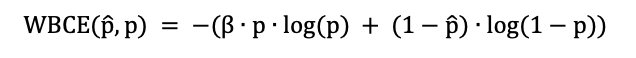The elbo in pytorch is divided into two parts, KL and reconstruction loss. My current dataset is a group of sparse binary matrices containing a small number of 1 and most of 0 . I want to use the weighted binary cross-entropy in the reconstruction loss and give a higher weight to the 1（Just like the formula in the picture, weight 1 value beta> 1 ）for better results in reconstruction, but I don’t know how to customize the elbo in pyro to achieve my goal.this is a pretty non-standard loss. so your best bet is probably to compute it by hand and use a pyro.factor statement in your model in place of the sample(..., obs=data) statement. something like:

my_log_loss = ...
pyro.factor("myfactor", -my_log_loss)  # with a minus sign because this goes into the ELBO

to do this correctly you need to pay attention to plates if your model uses plates.

1. Does the weighted binary cross entropy loss passed in via Pyro. factor replace Elbo’s original reconstruction loss? Because I want to.

2. Also I notice that torch.nn.BCELoss(weight=…) Can construct my target’s refactoring loss, I can apply pyro’s refactoring loss, can I do that?

3. If 2. doesn’t work, I have customized a reconstruction loss function that can achieve the purpose. How should I replace the reconstruction loss in ELbo?

def weighted_binary_cross_entropy(output, target, weights=None):
if weights is not None:
assert len(weights) == 2
loss = weights * (target * torch.log(output)) + \
weights * ((1 - target) * torch.log(1 - output))
else:
loss = target * torch.log(output) + (1 - target) * torch.log(1 - output)